parallel circuit and an open in a series circuit is that in the parallel circuit the open would not necessarily disable the circuit. If the open condition occurs in a series portion of the circuit, there will be no current because there is no complete path for current flow."> Effects of open and short circuitsCustom Search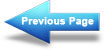Redrawing a Complex Circuit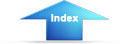Matter, Energy, and ElectricityVoltage dividersEFFECTS OF OPEN AND SHORT CIRCUITS
Earlier in this chapter the terms open and short circuits were discussed. The following discussion deals with the effects on a circuit when an open or a short occurs.

The major difference between an open in a parallel circuit and an open in a series circuit is that in the parallel circuit the open would not necessarily disable the circuit. If the open condition occurs in a series portion of the circuit, there will be no current because there is no complete path for current flow. If, on the other hand, the open occurs in a parallel path, some current will still flow in the circuit. The parallel branch where the open occurs will be effectively disabled, total resistance of the circuit will INCREASE, and total current will DECREASE.

To clarify these points, figure 3-61 illustrates a series parallel circuit. First the effect of an open in the series portion of this circuit will be examined. Figure 3-61(A) shows the normal circuit, RT = 40 ohms and IT = 3 amps. In figure 3-61(B) an open is shown in the series portion of the circuit, there is no complete path for current and the resistance of the circuit is considered to be infinite.

Figure 3-61. - Series-parallel circuit with opens.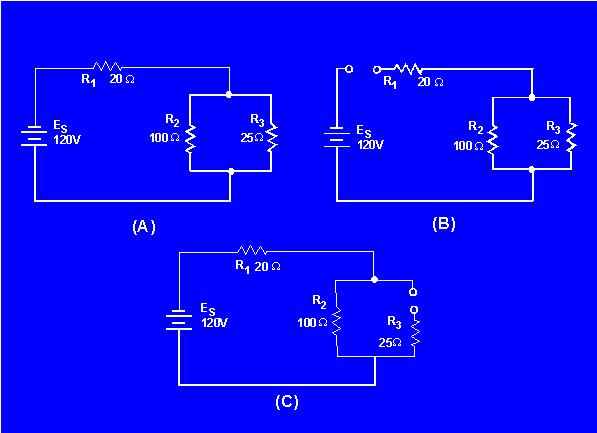In figure 3-61(C) an open is shown in the parallel branch of R3. There is no path for current through R3. In the circuit, current flows through R 1 and R2 only. Since there is only one path for current flow, R1 and R2 are effectively in series.

Under these conditions RT = 120Ω and IT = 1 amp. As you can see, when an open occurs in a parallel branch, total circuit resistance increases and total circuit current decreases.

A short circuit in a parallel network has an effect similar to a short in a series circuit. In general, the short will cause an increase in current and the possibility of component damage regardless of the type of circuit involved. To illustrate this point, figure 3-62 shows a series-parallel network in which shorts are developed. In figure 3-62 (A) the normal circuit is shown. RT = 40 ohms and I T = 3 amps.

Figure 3-62. - Series-parallel circuit with shorts.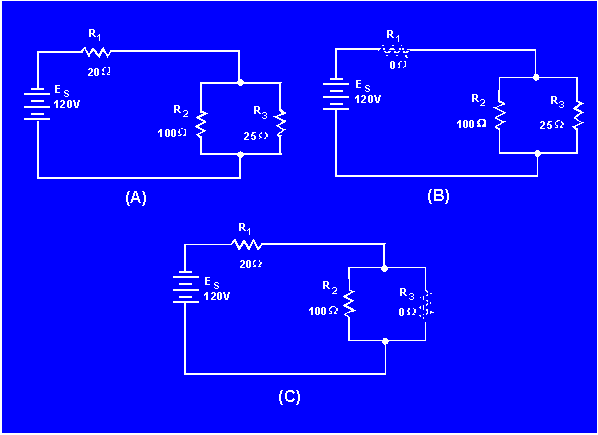In figure 3-62 (B), R1 has shorted. R1 now has zero ohms of resistance. The total of the resistance of the circuit is now equal to the resistance of the parallel network of R2 and R3, or 20 ohms. Circuit current has increased to 6 amps. All of this current goes through the parallel network (R2, R3) and this increase in current would most likely damage the components.

In figure 3-62 (C), R3 has shorted. With R3 shorted there is a short circuit in parallel with R2. The short circuit routes the current around R2, effectively removing R2 from the circuit. Total circuit resistance is now equal to the resistance of R1, or 20 ohms.

As you know, R2 and R3 form a parallel network. Resistance of the network can be calculated as follows:

Given: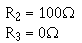Solution:The total circuit current with R3 shorted is 6 amps. All of this current flows through R1 and would most likely damage R1. Notice that even though only one portion of the parallel network was shorted, the entire paralleled network was disabled.

Opens and shorts alike, if occurring in a circuit, result in an overall change in the equivalent resistance. This can cause undesirable effects in other parts of the circuit due to the corresponding change in the total current flow. A short usually causes components to fail in a circuit which is not properly fused or otherwise protected. The failure may take the form of a burned-out resistor, damaged source, or a fire in the circuit components and wiring.

Fuses and other circuit protection devices are installed in equipment circuits to prevent damage caused by increases in current. These circuit protection devices are designed to open if current increases to a predetermined value. Circuit protection devices are connected in series with the circuit or portion of the circuit that the device is protecting. When the circuit protection device opens, current flow ceases in the circuit.

A more thorough explanation of fuses and other circuit protection devices is presented in Module 3, Introduction to Circuit Protection, Control, and Measurement.

What is the effect on total resistance and total current in a circuit if an open occurs in (a) a parallel branch, and (b) in a series portion?

What is the effect on total resistance and total current in a circuit if a short occurs in (a) a parallel branch, and (b) in a series portion?

If one branch of a parallel network is shorted, what portion of circuit current flows through the remaining branches?Integrated Publishing, Inc. - A (SDVOSB) Service Disabled Veteran Owned Small Business